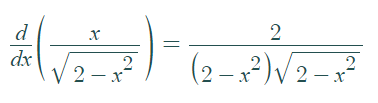# Bullet Nose Curve

A bullet nose curve is a quartic curve that gets its name because each half of the curve tapers to a pointed shape, just like the tip of a bullet.

The equation for a bullet nose curve, a special case of the Lamé curve, is
x2y2 – a2y2 + b2x2 = 0.
or, equivalently:
a2y2 – b2x2 = x2y2 or
y = ± bx / √(a2 – x2).
As a Cartesian parametrization: x = a cos t; y = b cot t.

The curve has three inflection points, where the curve changes from concave up to concave down (or vice versa). The curve has one singularity (called a node), two vertical asymptotes, two axes of symmetry, and three double points.

## Equation for a Tangent Line of a Bullet Nose Curve: Example

Example question: Find an equation for the tangent line at (1, 1) for the bullet nose curve y = |x| / √(2 – x2) .

Solution:
Step 1: Find the equation for the line. As x = 1 is positive, |x| becomes x. So the equation is:
y = x / √(2 – x2)

Step 2: Find the derivative of the equation from Step 1. I used Symbolab’s derivative calculator  to get:Step 3: Insert the x-value (from the question) into the equation for the first derivative (from Step 2). At (1, 1), the slope of the tangent line is f′(1) == 2.

Step 4: Insert your answer from Step 3 into the linear equation y = mx + b:
y = 2x + b.

Step 5: Figure out the “b” in the linear equation. This is where the line crosses the vertical axis. We know that the point (1, 1) lies on the tangent line, so we can substitute that in to find “b”:

• 1 = 2(1) + b.
• 1 = 2 + b
• b = -1

The tangent line crosses the y-axis at y = -1:This means that the equation is y = 2x -1.